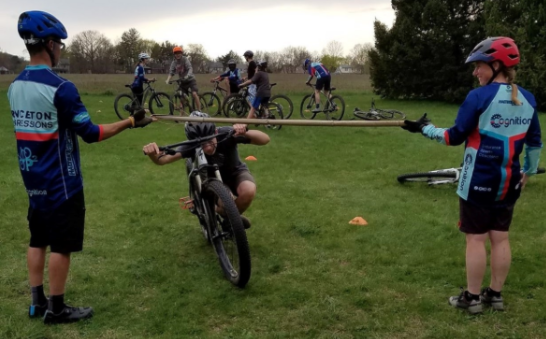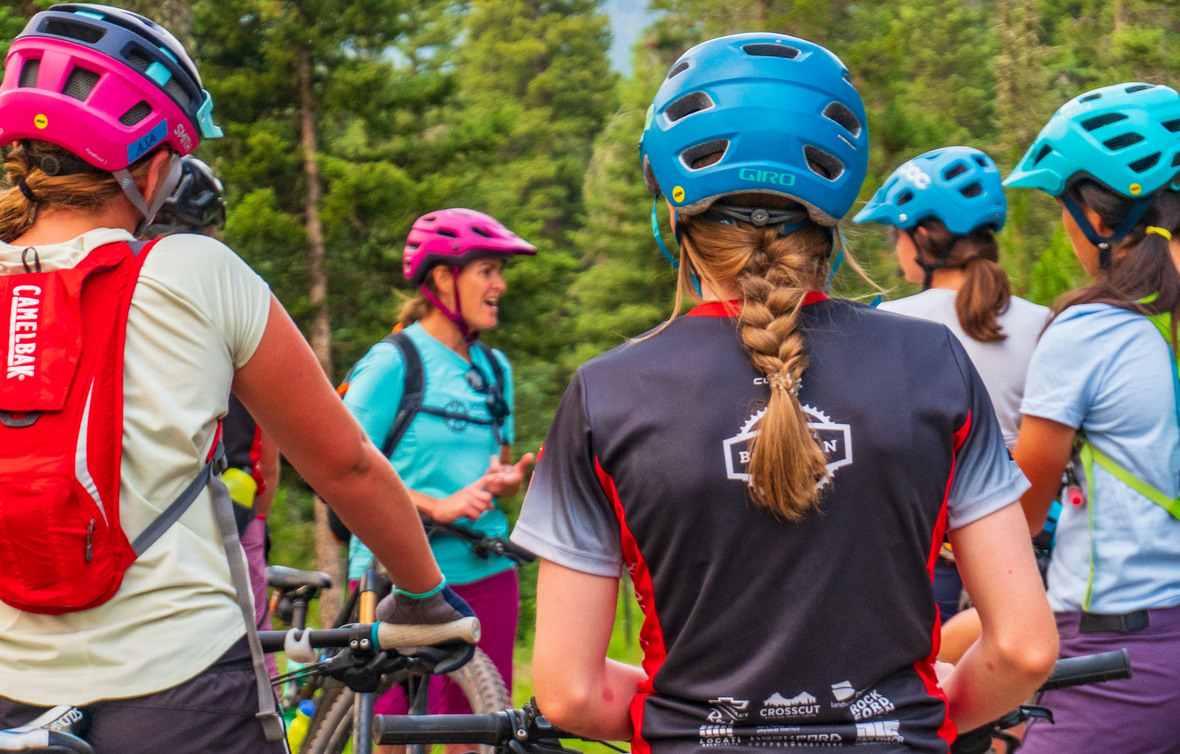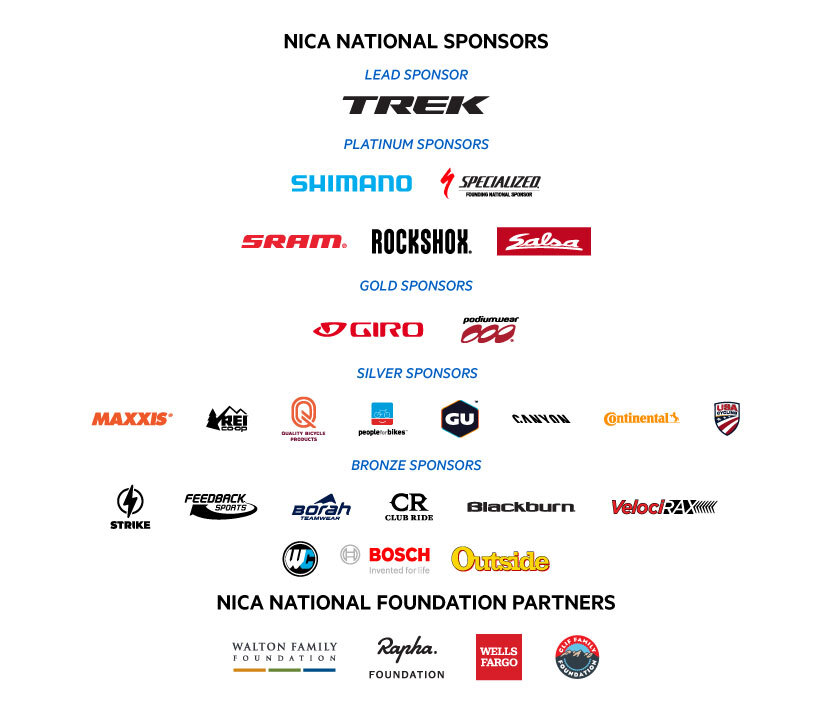Like   Tweet   Pin   +1   in
 /* styles */ Hello ! The fall 2022 season is around the corner and fall league coaches are starting their registration and training for the year. This is a good time to revisit your coach philosophy. Why do you coach? What is it like to be coached by you? What do you want it to be like to be coached by you? Take time to reflect on your philosophy or revise your philosophy. A solid Coach philosophy makes tough decisions easier and gives you a lens to frame everything you do as a coach. My Coach philosophy was inspired by Joe Ehrman. In his book Inside Out Coaching, he states his philosophy – “I coach to help young people become adults of empathy and integrity who will lead, be responsible, and change the world for good.” Sports are amazing and can help student-athletes discover things in themselves that they didn’t know were there before if they have the right coach to mentor them. Coaches Matter! Thanks for doing what you do. -Mike McGarry and the NICA Coach Licensing Team In This Issue: - Game of the Month: Bike Limbo - Fall 2022 Coaches Pit Zone Registration and Education - Online Leaders’ Summit - Over 2,000 Coaches certified! - Adventure Spotlight - GRiT Corner - New NICA CUEs available though COEUS - 2022 Sparkle On Scholarship - Trek NICA Pathfinders Scholarship - Applications due April 15th! - NICA Celebrates Four Years of Injury Reduction! - Partner and Sponsor Content - TrueSport: Coach’s Mental Health: How to Set Healthy Boundaries as a Coach
 table div table+table+table div table{width:100%;padding:0}table div table+table+table div table img{width:96.23%;padding:0;float:none}table div table+table+table div table td{width:100%;padding:0 1.88% 18px}/* styles */## Game of the Month - Bike LimboSend us images of your teams playing games! coachlicensing@nationalmtb.org

Equipment: Rope/Webbing/Pool Noodle
Where: Open Space
Objective: I can build timing, coordination, and pressure control skills. I can have fun.
Setup: Two coaches hold a long thin object such as rope, webbing, broom handle, or a rod of bamboo (for authenticity). Riders form a line and try to ride under one at a time.
101 Skills: Bike-Body Separation
Rules: Riders must ride under the height without touching the ground. Lower the object as riders progress. Riders are eliminated until you have a winner.

Reflection Question: What would you do differently next time?

 table div table+table+table+table+table+table div table{width:100%;padding:0}table div table+table+table+table+table+table div table img{width:96.23%;padding:0;float:none}table div table+table+table+table+table+table div table td{width:100%;padding:0 1.88% 18px}/* styles */## Fall 2022 Coaches Pit Zone Registration and Education

 table div table+table+table+table+table+table+table+table+table div table{width:100%;padding:0}table div table+table+table+table+table+table+table+table+table div table img{width:96.23%;padding:0;float:none}table div table+table+table+table+table+table+table+table+table div table td{width:100%;padding:0 1.88% 18px}/* styles */## Online Leaders’ Summit - Over 2,000 Coaches certified!

 table div table+table+table+table+table+table+table+table+table+table+table div table,table.module-10{width:48.11%;float:left;padding:0}table div table+table+table+table+table+table+table+table+table+table+table div table a{border:0 none;text-decoration:none}table div table+table+table+table+table+table+table+table+table+table+table div table img{width:100%!important;border:0 none;text-decoration:none}table div table+table+table+table+table+table+table+table+table+table+table div table td{width:100%;padding:0 20px 20px 0}/* styles */ In March 2020 the NICA Coach Licensing team saw a need to help coaches stay connected and continue their coach development education, while in-person training and events were being canceled, we explored new options in the virtual space. The first NICA National Online Leaders’ Summit took place in April 2020. The summits have evolved and many NICA coaches across the country have benefited from the convenience and camaraderie provided. In March of 2022 a milestone was hit when over 2,000 coaches completed their Leaders’ Summit requirement with the Online Summit. Thank you to all the amazing coaches that have attended. Your commitment and dedication helps get more kids on bikes. If you are interested in participating in a NICA National Online Leaders’ Summit you can find more information below: The Leaders’ Summit is a one time requirement for Level 3 (online or in person) and can also be used for CEUs for maintaining your Level 2 and 3 license. We award 1 CEU for each session, so if you attend all 5 you can receive 5 CEUs. If you are a Level 2 or 3 Coach looking for CEUs, the Online Leaders’ Summit is a great way to refresh your training while maintaining your license level. For the remainder of the 2022 schedule, registration, and information on upcoming Online Leaders’ Summits click the button below.
 table div table+table+table+table+table+table+table+table+table+table+table+table+table div table{width:100%;padding:0}table div table+table+table+table+table+table+table+table+table+table+table+table+table div table img{width:96.23%;padding:0;float:none}table div table+table+table+table+table+table+table+table+table+table+table+table+table div table td{width:100%;padding:0 1.88% 18px}/* styles */Every athlete is one relationship away from a successful life. - Joe Ehrmann

A huge part of quality coaching is developing positive relationships with athletes based on mutual trust and respect. Successful coaches have for a long time known that the time and energy invested in building quality relationships with their athletes pays huge dividends.

Ways You Can Build Relationships with Your Student-Athletes

▪ Making Time at the Right Time
Show up and make time for your student-athletes. Try to be available during informal times at practice; water breaks, activity transitions, before and after practice, etc. When talking with your student-athletes, square your shoulders and give them your full attention. Athletes may need your attention when it isn’t planned or timed perfectly, be sure to acknowledge their needs and make time.
▪ Get to Know Their Story
One of the foundations of building relationships is getting to know your athletes' stories. Show genuine interest in the young people you coach. Ask about their day. Asking the question “tell me about your day” will get a better response than, “how was your day?”
▪ Goal Setting
The process of setting team goals is a highly effective early season activity to build team cohesion. Early season goal setting for the athlete gives you some insight into what the athlete needs from you as a coach, and can be used as the launching point for an athlete-coach relationship. Both team and individual goal setting are more effective when followed up with regular check-ins.
▪ Creating Formal Time to Build Relationships
Intentionally set aside time to build relationships with your student-athletes through large group or small group check-ins.
▪ Check-in on team goals or ask about team cohesion - What have you done this week to support a teammate? What did you learn this week that you will use next week?
▪ Check--in on individual goals, or ask your athletes to reflect on practice - What went well this week? What can you improve? What did you learn this week that you will use next week?
▪ Community Service or Teen Trail Corps
Engaging your team in community service, trail maintenance, and stewardship is a great way to give back to the community and create an impactful shared experience.
 ▪ Making Time at the Right Time Show up and make time for your student-athletes. Try to be available during informal times at practice; water breaks, activity transitions, before and after practice, etc. When talking with your student-athletes, square your shoulders and give them your full attention. Athletes may need your attention when it isn’t planned or timed perfectly, be sure to acknowledge their needs and make time.
 ▪ Get to Know Their Story One of the foundations of building relationships is getting to know your athletes' stories. Show genuine interest in the young people you coach. Ask about their day. Asking the question “tell me about your day” will get a better response than, “how was your day?”
 ▪ Goal Setting The process of setting team goals is a highly effective early season activity to build team cohesion. Early season goal setting for the athlete gives you some insight into what the athlete needs from you as a coach, and can be used as the launching point for an athlete-coach relationship. Both team and individual goal setting are more effective when followed up with regular check-ins.
 ▪ Creating Formal Time to Build Relationships Intentionally set aside time to build relationships with your student-athletes through large group or small group check-ins.
 ▪ Check-in on team goals or ask about team cohesion - What have you done this week to support a teammate? What did you learn this week that you will use next week?
 ▪ Check--in on individual goals, or ask your athletes to reflect on practice - What went well this week? What can you improve? What did you learn this week that you will use next week?
 ▪ Community Service or Teen Trail Corps Engaging your team in community service, trail maintenance, and stewardship is a great way to give back to the community and create an impactful shared experience.table div table+table+table+table+table+table+table+table+table+table+table+table+table+table+table+table+table div table{width:100%;padding:0}table div table+table+table+table+table+table+table+table+table+table+table+table+table+table+table+table+table div table img{width:96.23%;padding:0;float:none}table div table+table+table+table+table+table+table+table+table+table+table+table+table+table+table+table+table div table td{width:100%;padding:0 1.88% 18px}/* styles */table div table+table+table+table+table+table+table+table+table+table+table+table+table+table+table+table+table+table div table td,table.module-17{width:100%;padding:0}table div table+table+table+table+table+table+table+table+table+table+table+table+table+table+table+table+table+table div table{width:100%;float:none;margin-left:auto;margin-right:auto;padding:0}table div table+table+table+table+table+table+table+table+table+table+table+table+table+table+table+table+table+table div table a{border:0 none;text-decoration:none}table div table+table+table+table+table+table+table+table+table+table+table+table+table+table+table+table+table+table div table img{width:100%!important;border:0 none;text-decoration:none}/* styles */
 /* styles */ Haley Tranum started as a new coach with the Arkansas League’s Lincoln Junior High team five years ago. When she started, there were just a couple of girls on her team. She wanted to support them, so she started leading ice cream rides – short trail rides ending at an ice cream shop, where they’d enjoy a treat and talk about life. The girls loved it and, over time, invited friends. The rides grew. This small activity, focused on community, relationships and fun, became a major draw for the team. Last season, Lincoln Junior High had the highest percentage of female student athletes of any team in Arkansas, at 35% (with the exception of one small team with 2 girls and 2 boys). And Haley agreed to share her experience and help grow GRiT across the League by serving as GRiT Coordinator. You can learn more about what Haley and the Arkansas League are doing to support female student athletes and coaches here: https://www.arkansasmtb.org/grit/ If you want to get more involved on your team, please reach out to your GRiT Coordinator! You can find a list here: https://www.nationalmtb.org/grit-coordinators/. Or email Emily Green, NICA GRiT Program Manager at egreen@nationalmtb.org and she’ll connect you with the right person in your League. Thanks for helping to get #moregirlsonbikes!
 table div table+table+table+table+table+table+table+table+table+table+table+table+table+table+table+table+table+table+table+table div table{width:100%;padding:0}table div table+table+table+table+table+table+table+table+table+table+table+table+table+table+table+table+table+table+table+table div table img{width:96.23%;padding:0;float:none}table div table+table+table+table+table+table+table+table+table+table+table+table+table+table+table+table+table+table+table+table div table td{width:100%;padding:0 1.88% 18px}/* styles */## New NICA CEUs available though COEUS

 table div table+table+table+table+table+table+table+table+table+table+table+table+table+table+table+table+table+table+table+table+table+table div table,table.module-21{width:47.17%;float:left;padding:0}table div table+table+table+table+table+table+table+table+table+table+table+table+table+table+table+table+table+table+table+table+table+table div table a{border:0 none;text-decoration:none}table div table+table+table+table+table+table+table+table+table+table+table+table+table+table+table+table+table+table+table+table+table+table div table img{width:100%!important;border:0 none;text-decoration:none}table div table+table+table+table+table+table+table+table+table+table+table+table+table+table+table+table+table+table+table+table+table+table div table td{width:100%;padding:0 20px 20px 0}/* styles */ Meet Coeus, a new education platform different from anything you’ve seen through NICA before. Co-founded by NICA coach-supporter Tim Curry, Coeus offers coaching education uniquely tailored to meet the needs of NICA coaches and teams. Our CEU courses were created in partnership with NICA, and offer immediately applicable information relevant to teaching youth athletes in areas including Nutrition, Skills and Performance. Each Coeus CEU course is certified by NICA for CEU credit and taught by experts in their field who actively teach at the collegiate level. Come discover our expanding library of courses with the tools to help you build strong coach-athlete relationships. Make your free account at www.coeus-ed.com to register for CEU courses, view free course previews, receive updates when new courses become available, and access all the free resources Coeus has to offer. We look forward to welcoming you to the Coeus community!

## 2022 Sparkle On Scholarship - Application Now Open until March 21st

 table div table+table+table+table+table+table+table+table+table+table+table+table+table+table+table+table+table+table+table+table+table+table+table+table div table,table.module-23{width:37.17%;float:left;padding:0}table div table+table+table+table+table+table+table+table+table+table+table+table+table+table+table+table+table+table+table+table+table+table+table+table div table a{border:0 none;text-decoration:none}table div table+table+table+table+table+table+table+table+table+table+table+table+table+table+table+table+table+table+table+table+table+table+table+table div table img{width:100%!important;border:0 none;text-decoration:none}table div table+table+table+table+table+table+table+table+table+table+table+table+table+table+table+table+table+table+table+table+table+table+table+table div table td{width:100%;padding:0 20px 20px 0}/* styles */ The Sparkle On Scholarship presented by Kate Courtney in partnership with SCOTT, Syncros, SRAM, and RockShox recognizes and supports student-athletes who have demonstrated a commitment to education and the cycling community during their time with NICA and will soon attend college. This year, Sparkle On will be awarded to six NICA seniors who show determination in pursuit of academic and athletic goals and a commitment to the cycling community and intend to continue to support and participate in cycling in college. The scholarships will include a monetary award of \$6,500 each, mentorship in the form of quarterly calls with Kate, and participation in a Summit focused on skill building, on and off the bike. If you know a senior who would be a good fit then direct them here to apply. Please note that we may also reach out to coaches the week of 15th-22nd April for references should a student-athlete on your team make the list of semi-finalists.

## Trek NICA Pathfinders Scholarship - Application Deadline April 15!

 table div table+table+table+table+table+table+table+table+table+table+table+table+table+table+table+table+table+table+table+table+table+table+table+table+table+table+table div table,table.module-26{width:32.08%;float:right;padding:0}table div table+table+table+table+table+table+table+table+table+table+table+table+table+table+table+table+table+table+table+table+table+table+table+table+table+table+table div table a{border:0 none;text-decoration:none}table div table+table+table+table+table+table+table+table+table+table+table+table+table+table+table+table+table+table+table+table+table+table+table+table+table+table+table div table img{width:100%!important;border:0 none;text-decoration:none}table div table+table+table+table+table+table+table+table+table+table+table+table+table+table+table+table+table+table+table+table+table+table+table+table+table+table+table div table td{width:100%;padding:0 0 20px 20px}/* styles */ We are excited to announce that applications are now open for Trek NICA Pathfinders Scholarships for our Fall Leagues! Trek has partnered with NICA to grow diversity in the sport of cycling and provide better access for people of color. This scholarship will provide up to 250 diverse and underrepresented youth with the gear and funds they need to ride and race in their local NICA League. If you know a student-athlete who would be a good fit, learn more and apply here! The deadline to apply is April 15! See the story of Genesis, a Park City, Utah teen and Pathfinders recipient whose life has been transformed through greater access to the outdoors, here.

## NICA Celebrates Four Years of Injury Reduction!

 /* styles */ Since 2018 when NICA and the University of Utah launched the NICA Safety Reporting and Study Partnership, we’ve seen an injury-rate reduction for Student-Athletes by 38% and Coaches by 25%. While injuries are inherent to participation in NICA as with any sports organization, we strive to minimize potential for injuries. To continue on our path of injury reduction, please keep practicing fundamental skills among other safety best practices.
 table div table+table+table+table+table+table+table+table+table+table+table+table+table+table+table+table+table+table+table+table+table+table+table+table+table+table+table+table+table+table div table td,table.module-29{width:100%;padding:0}table div table+table+table+table+table+table+table+table+table+table+table+table+table+table+table+table+table+table+table+table+table+table+table+table+table+table+table+table+table+table div table{width:100%;float:none;margin-left:auto;margin-right:auto;padding:0}table div table+table+table+table+table+table+table+table+table+table+table+table+table+table+table+table+table+table+table+table+table+table+table+table+table+table+table+table+table+table div table a{border:0 none;text-decoration:none}table div table+table+table+table+table+table+table+table+table+table+table+table+table+table+table+table+table+table+table+table+table+table+table+table+table+table+table+table+table+table div table img{width:100%!important;border:0 none;text-decoration:none}/* styles */
 table div table+table+table+table+table+table+table+table+table+table+table+table+table+table+table+table+table+table+table+table+table+table+table+table+table+table+table+table+table+table+table div table{width:100%;padding:0}table div table+table+table+table+table+table+table+table+table+table+table+table+table+table+table+table+table+table+table+table+table+table+table+table+table+table+table+table+table+table+table div table img{width:96.23%;padding:0;float:none}table div table+table+table+table+table+table+table+table+table+table+table+table+table+table+table+table+table+table+table+table+table+table+table+table+table+table+table+table+table+table+table div table td{width:100%;padding:0 1.88% 18px}/* styles */## NICA Partners and Sponsors

 table div table+table+table+table+table+table+table+table+table+table+table+table+table+table+table+table+table+table+table+table+table+table+table+table+table+table+table+table+table+table+table+table+table div table,table.module-32{width:40.75%;float:left;padding:0}table div table+table+table+table+table+table+table+table+table+table+table+table+table+table+table+table+table+table+table+table+table+table+table+table+table+table+table+table+table+table+table+table+table div table a{border:0 none;text-decoration:none}table div table+table+table+table+table+table+table+table+table+table+table+table+table+table+table+table+table+table+table+table+table+table+table+table+table+table+table+table+table+table+table+table+table div table img{width:100%!important;border:0 none;text-decoration:none}table div table+table+table+table+table+table+table+table+table+table+table+table+table+table+table+table+table+table+table+table+table+table+table+table+table+table+table+table+table+table+table+table+table div table td{width:100%;padding:0 20px 20px 0}/* styles */ Trek makes up the largest annual contribution to the National Interscholastic Cycling Association. They’ve committed to helping NICA get more kids on bikes because they’ve seen firsthand how mountain biking can be a positive force for every rider. As a student-athlete, you’re eligible for great discounts on Trek bikes and Bontrager gear that can help you make the most of your season. www.trekbikes.com
 table div table+table+table+table+table+table+table+table+table+table+table+table+table+table+table+table+table+table+table+table+table+table+table+table+table+table+table+table+table+table+table+table+table+table div table{width:100%;padding:0}table div table+table+table+table+table+table+table+table+table+table+table+table+table+table+table+table+table+table+table+table+table+table+table+table+table+table+table+table+table+table+table+table+table+table div table img{width:96.23%;padding:0;float:none}table div table+table+table+table+table+table+table+table+table+table+table+table+table+table+table+table+table+table+table+table+table+table+table+table+table+table+table+table+table+table+table+table+table+table div table td{width:100%;padding:0 1.88% 18px}/* styles */table div table+table+table+table+table+table+table+table+table+table+table+table+table+table+table+table+table+table+table+table+table+table+table+table+table+table+table+table+table+table+table+table+table+table+table div table,table.module-34{width:29.43%;float:right;padding:0}table div table+table+table+table+table+table+table+table+table+table+table+table+table+table+table+table+table+table+table+table+table+table+table+table+table+table+table+table+table+table+table+table+table+table+table div table a{border:0 none;text-decoration:none}table div table+table+table+table+table+table+table+table+table+table+table+table+table+table+table+table+table+table+table+table+table+table+table+table+table+table+table+table+table+table+table+table+table+table+table div table img{width:100%!important;border:0 none;text-decoration:none}table div table+table+table+table+table+table+table+table+table+table+table+table+table+table+table+table+table+table+table+table+table+table+table+table+table+table+table+table+table+table+table+table+table+table+table div table td{width:100%;padding:0 0 20px 20px}/* styles */ Planet Bike believes that life is better by bike, which is why they are dedicated to creating products that help make cycling a daily part of people’s lives! Planet Bike’s products are focused on sustainability, and their business is driven by the belief that the world would be a better place if everyone spent a little more time on their bikes! We at NICA agree, and appreciate their sponsorship to help us make cycling a vital part of our student-athletes’ lives! www.planetbike.com
 table div table+table+table+table+table+table+table+table+table+table+table+table+table+table+table+table+table+table+table+table+table+table+table+table+table+table+table+table+table+table+table+table+table+table+table+table div table{width:100%;padding:0}table div table+table+table+table+table+table+table+table+table+table+table+table+table+table+table+table+table+table+table+table+table+table+table+table+table+table+table+table+table+table+table+table+table+table+table+table div table img{width:96.23%;padding:0;float:none}table div table+table+table+table+table+table+table+table+table+table+table+table+table+table+table+table+table+table+table+table+table+table+table+table+table+table+table+table+table+table+table+table+table+table+table+table div table td{width:100%;padding:0 1.88% 18px}/* styles */table div table+table+table+table+table+table+table+table+table+table+table+table+table+table+table+table+table+table+table+table+table+table+table+table+table+table+table+table+table+table+table+table+table+table+table+table+table div table,table.module-36{width:37.92%;float:left;padding:0}table div table+table+table+table+table+table+table+table+table+table+table+table+table+table+table+table+table+table+table+table+table+table+table+table+table+table+table+table+table+table+table+table+table+table+table+table+table div table a{border:0 none;text-decoration:none}table div table+table+table+table+table+table+table+table+table+table+table+table+table+table+table+table+table+table+table+table+table+table+table+table+table+table+table+table+table+table+table+table+table+table+table+table+table div table img{width:100%!important;border:0 none;text-decoration:none}table div table+table+table+table+table+table+table+table+table+table+table+table+table+table+table+table+table+table+table+table+table+table+table+table+table+table+table+table+table+table+table+table+table+table+table+table+table div table td{width:100%;padding:0 20px 20px 0}/* styles */ What are the keys to warmth for mid-winter riding? According to Fox, there are two important steps to keeping cozy in the cold: first, dial in your base with thermo layers, then insulate with breathable outer layers that combat the elements! Make sure to check out Fox's biking gear. Keep warm and bike on! www.foxracing.com
 table div table+table+table+table+table+table+table+table+table+table+table+table+table+table+table+table+table+table+table+table+table+table+table+table+table+table+table+table+table+table+table+table+table+table+table+table+table+table div table{width:100%;padding:0}table div table+table+table+table+table+table+table+table+table+table+table+table+table+table+table+table+table+table+table+table+table+table+table+table+table+table+table+table+table+table+table+table+table+table+table+table+table+table div table img{width:96.23%;padding:0;float:none}table div table+table+table+table+table+table+table+table+table+table+table+table+table+table+table+table+table+table+table+table+table+table+table+table+table+table+table+table+table+table+table+table+table+table+table+table+table+table div table td{width:100%;padding:0 1.88% 18px}/* styles */table div table+table+table+table+table+table+table+table+table+table+table+table+table+table+table+table+table+table+table+table+table+table+table+table+table+table+table+table+table+table+table+table+table+table+table+table+table+table+table div table,table.module-38{width:42.26%;float:right;padding:0}table div table+table+table+table+table+table+table+table+table+table+table+table+table+table+table+table+table+table+table+table+table+table+table+table+table+table+table+table+table+table+table+table+table+table+table+table+table+table+table div table a{border:0 none;text-decoration:none}table div table+table+table+table+table+table+table+table+table+table+table+table+table+table+table+table+table+table+table+table+table+table+table+table+table+table+table+table+table+table+table+table+table+table+table+table+table+table+table div table img{width:100%!important;border:0 none;text-decoration:none}table div table+table+table+table+table+table+table+table+table+table+table+table+table+table+table+table+table+table+table+table+table+table+table+table+table+table+table+table+table+table+table+table+table+table+table+table+table+table+table div table td{width:100%;padding:0 0 20px 20px}/* styles */ We are excited to have a partnership with Specialized! As a leading bike manufacturer and founding NICA sponsor, Specialized understands how important it is to get more kids on bikes and supports our mission to build strong minds, bodies, character and communities. www.specialized.com
 table div table+table+table+table+table+table+table+table+table+table+table+table+table+table+table+table+table+table+table+table+table+table+table+table+table+table+table+table+table+table+table+table+table+table+table+table+table+table+table+table div table{width:100%;padding:0}table div table+table+table+table+table+table+table+table+table+table+table+table+table+table+table+table+table+table+table+table+table+table+table+table+table+table+table+table+table+table+table+table+table+table+table+table+table+table+table+table div table img{width:96.23%;padding:0;float:none}table div table+table+table+table+table+table+table+table+table+table+table+table+table+table+table+table+table+table+table+table+table+table+table+table+table+table+table+table+table+table+table+table+table+table+table+table+table+table+table+table div table td{width:100%;padding:0 1.88% 18px}/* styles */table div table+table+table+table+table+table+table+table+table+table+table+table+table+table+table+table+table+table+table+table+table+table+table+table+table+table+table+table+table+table+table+table+table+table+table+table+table+table+table+table+table div table,table.module-40{width:24.91%;float:left;padding:0}table div table+table+table+table+table+table+table+table+table+table+table+table+table+table+table+table+table+table+table+table+table+table+table+table+table+table+table+table+table+table+table+table+table+table+table+table+table+table+table+table+table div table a{border:0 none;text-decoration:none}table div table+table+table+table+table+table+table+table+table+table+table+table+table+table+table+table+table+table+table+table+table+table+table+table+table+table+table+table+table+table+table+table+table+table+table+table+table+table+table+table+table div table img{width:100%!important;border:0 none;text-decoration:none}table div table+table+table+table+table+table+table+table+table+table+table+table+table+table+table+table+table+table+table+table+table+table+table+table+table+table+table+table+table+table+table+table+table+table+table+table+table+table+table+table+table div table td{width:100%;padding:0 20px 20px 0}/* styles */ Thank You to Worldwide Cyclery for continuing to support NICA as a sponsor in 2022. They’re here to support people having fun on bikes, and that means more kids on bikes too. AND as part of a friendly staff competition, they rode a combined total of 30,380 miles! For every mile ridden, Worldwide Cyclery donated to NICA, which ended up to total almost \$20,000 (on top of their annual donation!) We cannot thank them enough! www.worldwidecylery.com
 table div table+table+table+table+table+table+table+table+table+table+table+table+table+table+table+table+table+table+table+table+table+table+table+table+table+table+table+table+table+table+table+table+table+table+table+table+table+table+table+table+table+table div table{width:100%;padding:0}table div table+table+table+table+table+table+table+table+table+table+table+table+table+table+table+table+table+table+table+table+table+table+table+table+table+table+table+table+table+table+table+table+table+table+table+table+table+table+table+table+table+table div table img{width:96.23%;padding:0;float:none}table div table+table+table+table+table+table+table+table+table+table+table+table+table+table+table+table+table+table+table+table+table+table+table+table+table+table+table+table+table+table+table+table+table+table+table+table+table+table+table+table+table+table div table td{width:100%;padding:0 1.88% 18px}/* styles */## TrueSport: Coach’s Mental Health: How to Set Healthy Boundaries as a Coach

 table div table+table+table+table+table+table+table+table+table+table+table+table+table+table+table+table+table+table+table+table+table+table+table+table+table+table+table+table+table+table+table+table+table+table+table+table+table+table+table+table+table+table+table+table+table+table+table div table{width:100%;padding:0}table div table+table+table+table+table+table+table+table+table+table+table+table+table+table+table+table+table+table+table+table+table+table+table+table+table+table+table+table+table+table+table+table+table+table+table+table+table+table+table+table+table+table+table+table+table+table+table div table img{width:96.23%;padding:0;float:none}table div table+table+table+table+table+table+table+table+table+table+table+table+table+table+table+table+table+table+table+table+table+table+table+table+table+table+table+table+table+table+table+table+table+table+table+table+table+table+table+table+table+table+table+table+table+table+table div table td{width:100%;padding:0 1.88% 18px}/* styles */